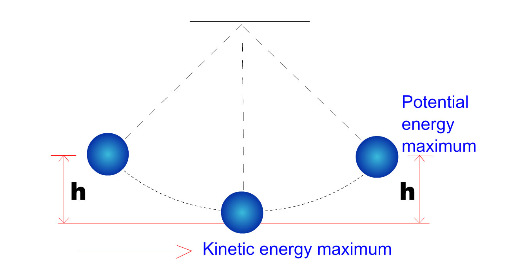To demonstrate Interconversion of Potential and Kinetic Energy

# Our Objective

To demonstrate interconversion of potential and kinetic energy.

# The Theory

Energy is the ability to do work or cause change. Energy comes in two basic types: kinetic energy and potential energy. Kinetic energy is the energy an object has because of its motion. It depends on the object’s mass and velocity. Potential energy is the energy that results from the position or shape of an object.

Mechanical energy is the sum of the kinetic and potential energies in a system. The principle of the conservation of energy states that the total mechanical energy in a system is the sum of the potential and the kinetic energies which remain constant if the forces which are acting are conservative forces.

How are Potential and Kinetic Energy Related?
When an object moves, potential energy transforms into kinetic energy. An object at rest has potential energy but no kinetic energy. Mechanical energy refers to the sum of both kinetic and potential energy.

In experiment, the wheel gains some potential energy as it rises. When released, it falls, and its potential energy is changed into the kinetic energy of the wheel's rotation.# Learning Outcomes

• Students can understand the term ‘Energy’.
• Students can understand the two types of energy and how they act.
• Students are better able to perform the experiment in the real lab after experiencing it in the virtual lab.